Main Content

# 5G NR Downlink Carrier Aggregation, Demodulation, and Analysis

This example shows how to generate, aggregate, and demodulate multiple downlink carriers using 5G Toolbox™ features.

### Introduction

This example generates an NR waveform with carrier aggregation (CA). To perform carrier aggregation, the example calculates the frequency offsets for the intraband contiguous CA case, as described in TS 38.104 Section 5.3A. The example also supports customized intraband noncontiguous and interband CA scenarios.

To generate an aggregated downlink waveform, the example configures a standard-compliant downlink fixed reference channel (FRC) for each component carrier. TS 38.101-1 Annex A.3 defines the physical downlink shared channel (PDSCH) FRCs for FR1 and TS 38.101-2 Annex A.3 defines the PDSCH FRCs for FR2. For the FRC waveform generation, you can specify the channel bandwidth, the subcarrier spacing, the modulation, the duplexing mode, and the cell ID. For more information on how to generate DL FRCs, see 5G NR-TM and FRC Waveform Generation.

After generating the component carriers (CCs), the example resamples the waveforms to a common sample rate and combines the CCs to generate the aggregated waveform.

Finally, the example filters and downsamples a selected CC to perform EVM measurements and PDSCH decoding.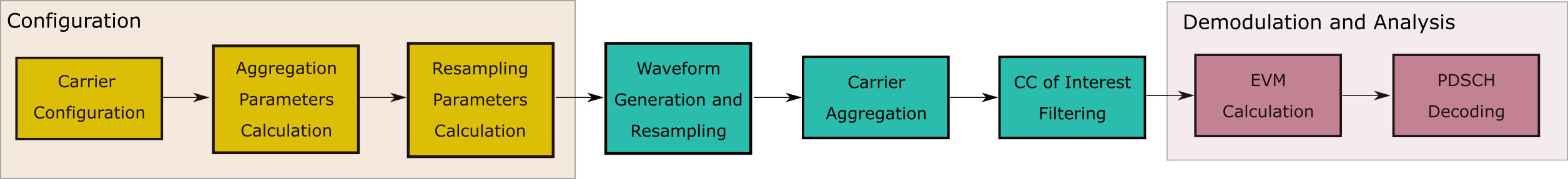### Selection of Component Carrier Parameters

The vector `ChannelBandwidths` specifies the bandwidth for each CC. The length of this vector corresponds to the number of CCs. The elements of `ChannelBandwidths` must be in the set {5, 10, 15, 20, 25, 30, 40, 50, 60, 70, 80, 90 100} MHz for FR1 and in the set {50, 100, 200, 400} MHz for FR2. TS 38.101-1 Section 5.5A.1 Table 5.5A.1-1 and TS 38.101-2 Section 5.5A.1 Table 5.5A.1-1 list the valid combinations of bandwidths for FR1 and FR2 carrier aggregation, respectively.

If the `ccSpacings` vector is empty, the example calculates the spacings between consecutive carriers, `ccSpacings`, as described in TS 38.104. To select the spacings of your choice, add the spacings to the `ccSpacings` vector.

```% Configure three carriers for the aggregation. You can select a different % number of carriers by modifying the number of elements in the % |channelBandwidths|, |SCSs|, |modulations|, and |nCellIDs| vectors. frequencyRange = 'FR1'; % (FR1 or FR2) channelRaster = 100; % Channel raster in kHz (15, 60 or 100) channelBandwidths = [60 40 40]; % Channel bandwidths in MHz % (5, 10, 15, 20, 25, 30, 40, 50, 60, 70, 80, % 90 or 100 for FR1) % (50, 100, 200 or 400 for FR2) SCSs = [30 30 30]; % Subcarrier spacings in kHz % (15, 30 or 60 for FR1) % (60 or 120 for FR2) modulations = {'QPSK' '64QAM' '256QAM'}; % ('QPSK', '64QAM' or '256QAM' for FR1) % ('QPSK', '16QAM' or '64QAM' for FR2) nCellIDs = [1 2 3]; % Cell IDs duplexMode = 'TDD'; % Duplex mode ('TDD' or 'FDD') sv = '15.2.0'; % Standard version ('15.1.0', '15.2.0' or '15.7.0') numSubframes = 10; % Number of subframes to generate per carrier % Choose the spacings between consecutive carriers, |ccSpacings|, or leave % the vector empty for calculating the spacings, as described in TS 38.104 % Section 5.3A ccSpacings = []; % MHz % Select the CC to demodulate CCofInterest = 2; % Verify the number of CCs, channel bandwidths, subcarrier spacings, % modulations, and spacings. Additionally, check that the spacings are % greater than 0. hNRVerifyCarrierParameters(length(channelBandwidths),length(SCSs),... length(modulations),length(nCellIDs),ccSpacings);```

### Component Carrier Configuration

Generate a configuration structure for each CC using `hNRReferenceWaveformGenerator`. Store the configuration structures for all CCs in a cell array.

```% Establish the number of component carriers numCC = length(channelBandwidths); % CC configuration referenceChannel = cell(1,numCC); wavegen = cell(1,numCC); for i = 1:numCC % Select a reference channel, FRC, based on the chosen modulation and % frequency range referenceChannel{i} = strcat('DL-FRC-',frequencyRange,'-',modulations{i}); % Create a generator object for the above PDSCH FRC reference channel wavegen{i} = hNRReferenceWaveformGenerator(referenceChannel{i},... channelBandwidths(i),SCSs(i),duplexMode,nCellIDs(i),sv); end```

### Carrier Aggregation Parameters Calculation

To perform the carrier aggregation, you must calculate the frequency parameters described in TS 38.104, Sections 5.3 and 5.4.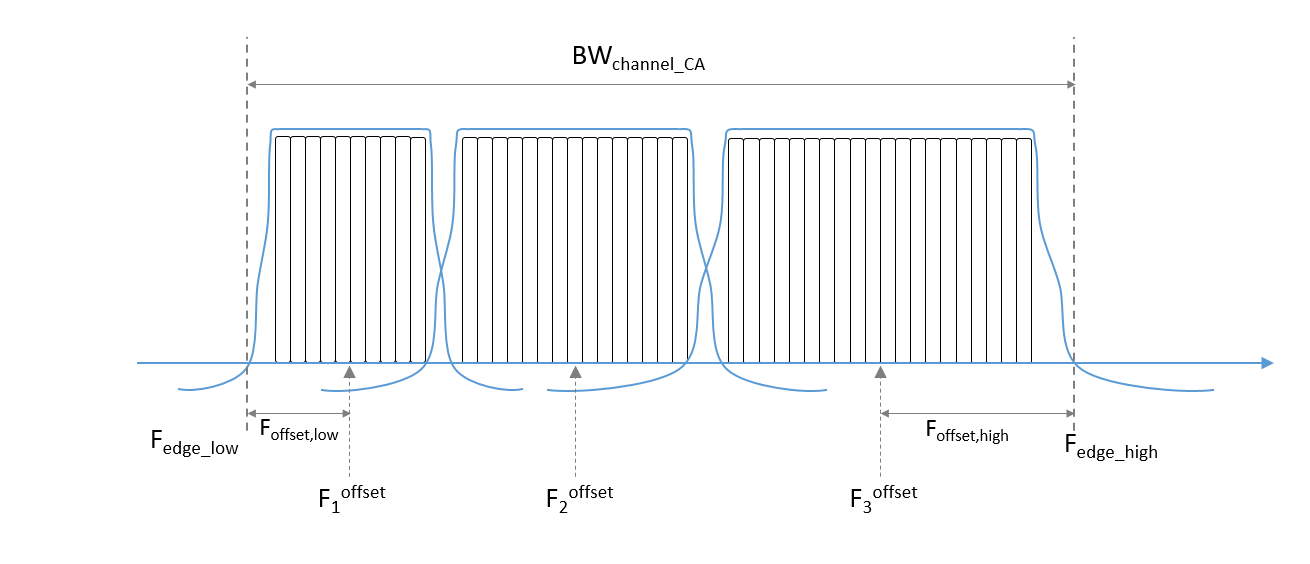• `Fc_offset` is a vector containing the center frequency of each CC at baseband

• `F_offset_low` is the frequency offset from the lower `Fc_offset` to the lower aggregated bandwidth edge

• `F_offset_high` is the frequency offset from the upper `Fc_offset` to the upper aggregated channel bandwidth edge

• `F_edge_low` is the lower edge of the aggregated channel bandwidth

• `F_edge_high` is the upper edge of the aggregated channel bandwidth

• `BW_channel_CA` is the aggregated channel bandwidth of all CCs

Center the lower carrier component at baseband (`Fc_offset(1)` = 0 Hz) and compute the center frequency for the rest of the CCs. To determine the center frequencies by following the method described in TS 38.104 Section 5.3A, you must calculate the minimum guardbands and the minimum CC spacings. Alternatively, to select your own center frequencies, choose a nonempty `ccSpacings` vector.

```% Get the largest SCS configuration, |SCSConfig|, among the SCS % configurations supported for each two consecutive channel bandwidths to % obtain the minimum guardband, as described in TS 38.104 Section 5.4.1.2. table = hGetGBTable(frequencyRange); % Minimum guardband table if isequal(frequencyRange,'FR1') if any(channelBandwidths(:) == 5) SCSConfig = 1; else SCSConfig = 2; end else SCSConfig = 3; end % Calculate the minimum guardband, as described in TS 38.104 Section 5.3.3 % Table 5.3.3-1 for FR1 and Table 5.3.3-1 for FR2, to obtain the minimum CC % spacings. minimumGuardBand = zeros(1,numCC); NDLRB = zeros(1,numCC); scs = strcat(num2str(2^SCSConfig*15),'kHz'); % Common SCS in kHz to extract % minimum guardband for i = 1:numCC NDLRB(i) = wavegen{i}.Config.SCSCarriers{1}.NSizeGrid; minimumGuardBand(i) = table{{scs},{strcat(num2str(channelBandwidths(i)),... 'MHz');}}; % kHz minimumGuardBand(i) = minimumGuardBand(i)*1e-3; % MHz end % Compute the minimum CC spacings, as defined in TS 38.104 Section % 5.4.1.2. Use these spacings to calculate the center frequencies and for % filtering each CC. minCCSpacings = zeros(1,numCC-1); % Minimum CC spacing for k = 2:numCC minCCSpacings(k-1) = hNRCarrierAggregationChannelSpacing( ... channelBandwidths(k-1), channelBandwidths(k), minimumGuardBand(k-1), ... minimumGuardBand(k),channelRaster,SCSConfig); % MHz end % Determine the center frequency for each CC with respect to 0 Hz. % Initially, the lower carrier frequency is at baseband (Fc_offset(1) = 0 Hz). Fc_offset = zeros(1,numCC); if isempty(ccSpacings) ccSpacings = minCCSpacings; end for k = 2:numCC Fc_offset(k) = Fc_offset(k-1) + ccSpacings(k-1); % MHz end```

Calculate the frequency offsets from the lower and upper center frequencies to the lower and upper aggregated bandwidth edges, respectively, as described in TS 38.104 Section 5.3A.

```F_offset_low = (NDLRB(1)*12+1)*(SCSs(1)*1e-3)/2 + minimumGuardBand(1); % MHz F_offset_high = (NDLRB(end)*12-1)*(SCSs(end)*1e-3)/2 + minimumGuardBand(end); %MHz```

Compute the lower and upper edges of the aggregated channel bandwidth, TS 38.104 Section 5.3A.

```F_edge_low = Fc_offset(1) - F_offset_low; % MHz F_edge_high = Fc_offset(end) + F_offset_high; % MHz```

Calculate the aggregated channel bandwidth, TS 38.104 Section 5.3A

```BW_channel_CA = F_edge_high - F_edge_low; % MHz fprintf('BW_channel_CA: %0.4f MHz\n',BW_channel_CA);```
```BW_channel_CA: 141.0800 MHz ```

Determine the frequency shift to center the aggregated channel bandwidth at baseband (0 Hz).

```shiftToCenter = -1*(BW_channel_CA/2 + F_edge_low); % Center aggregated bandwidth at baseband Fc_offset = Fc_offset + shiftToCenter; F_edge_low = Fc_offset(1) - F_offset_low; F_edge_high = Fc_offset(end) + F_offset_high; % Display frequency band edges fprintf('F_edge_low: %0.4f MHz\n',F_edge_low);```
```F_edge_low: -70.5400 MHz ```
`fprintf('F_edge_high: %0.4f MHz\n',F_edge_high);`
```F_edge_high: 70.5400 MHz ```
`fprintf('F_offset_low: %0.4f MHz\n',F_offset_low);`
```F_offset_low: 30.7050 MHz ```
`fprintf('F_offset_high: %0.4f MHz\n',F_offset_high);`
```F_offset_high: 20.6750 MHz ```
```% Display carrier frequencies fprintf('\n'); for i = 1:numCC fprintf('Component Carrier %d:\n',i); fprintf(' Fc: %0.4f MHz\n', Fc_offset(i)); end```
```Component Carrier 1: ```
``` Fc: -39.8350 MHz ```
```Component Carrier 2: ```
``` Fc: 9.9650 MHz ```
```Component Carrier 3: ```
``` Fc: 49.8650 MHz ```

### Oversampling Rate Calculation

Calculate the required oversampling factors for each component carrier, `OSRs`, to use a common sampling rate for the aggregated waveform.

```% Obtain sample rates of the component carriers CCSR = zeros(1,numCC); carriers = cell(1,numCC); for i = 1:numCC carriers{i} = nrCarrierConfig; carriers{i}.NCellID = nCellIDs(i); carriers{i}.NSizeGrid = NDLRB(i); carriers{i}.SubcarrierSpacing = SCSs(i); carriers{i}.CyclicPrefix = wavegen{i}.Config.BandwidthParts{1}.CyclicPrefix; info = nrOFDMInfo(carriers{i}); CCSR(i) = info.SampleRate; % Hz end % Calculate the oversampling ratio for the largest BW CC to ensure the % waveform occupies a maximum of 85% of the total bandwidth, |bwfraction| bwfraction = 0.85; % Bandwidth utilization of 85% OSR = (BW_channel_CA/bwfraction)/(max(CCSR)/1e6); % To simplify the resampling operation, choose an oversampling ratio which % is a power of 2: calculate the next power of two above OSR OSR = 2^ceil(log2(OSR)); % Calculate the overall sample rate for the aggregated waveform SR = OSR*max(CCSR); % Hz fprintf('\nOutput sample rate: %0.4f Ms/s\n\n',SR/1e6);```
```Output sample rate: 245.7600 Ms/s ```
```% Calculate the individual oversampling factors for the component carriers OSRs = SR./CCSR;```

### Waveform Generation and Carrier Aggregation

Call the `generateWaveform` function from the `hNRReferenceWaveformGenerator` helper file to generate the waveform for each CC. Resample each carrier to a common sample rate, frequency modulate the carriers to the appropriate center frequency, and finally aggregate the CCs to form the combined waveform.

```% Generate and aggregate the component carriers waveform = 0; tmwaveinfo = cell(1,numCC); waveInfo = cell(1,numCC); resourcesInfo = cell(1,numCC); frequencyShifter = comm.PhaseFrequencyOffset('SampleRate',SR); for i = 1:numCC % Generate each CC [wf,waveInfo{i},resourcesInfo{i}] = generateWaveform(wavegen{i},... numSubframes); % Resample the CCs so that they have the same sample rate wf = resample(wf,OSRs(i),1)/OSRs(i); % Frequency modulate each CC to the appropiate center frequency frequencyShifter.FrequencyOffset = Fc_offset(i)*1e6; wf = frequencyShifter(wf); frequencyShifter.release(); % Aggregate the CCs to form the combined signal waveform = waveform + wf; end```

### Plot Carrier Aggregated Spectrum

Display the spectrum of the carrier aggregated signal by using the `hNRCarrierAggregationPlotSpectrum` helper function. The spectrum plot shows the individual carrier bandwidths. This example does not upconvert the waveform to radio frequency (RF), the center of the aggregated bandwidth is at baseband (0 Hz).

```specPlot = hNRCarrierAggregationPlotSpectrum(waveform,SR,... 'Power Spectrum of Carrier Aggregation',{'Signal spectrum'});```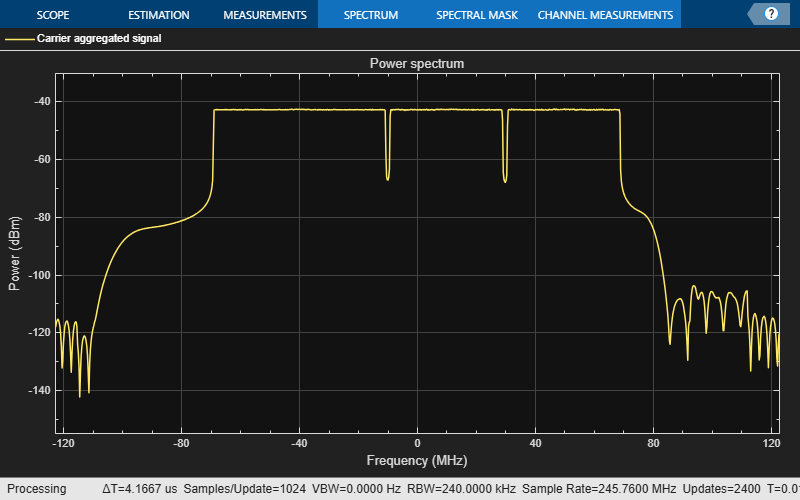### CC Demodulation and Filtering

Choose a CC, then demodulate, filter and downsample the CC of your choice, following these steps.

• Bring the CC to baseband (0 Hz)

• Calculate the passband and stopband frequencies of the filter

• Filter out the neighboring CCs by using the designed filter and downsample the CC.

```% Verify carrier of interest ID if CCofInterest > numCC || CCofInterest <= 0 || mod(CCofInterest,1) ~= 0 error('nr5g:NRDownlinkCarrierAggregationExample:CCOutOfRange',... 'Cannot demodulate CC number %d, choose an integer number that falls between 1 and %d\n',... CCofInterest, numCC) ; end fprintf(1,'Extracting CC number %d: \n', CCofInterest);```
```Extracting CC number 2: ```
```% Define downsampling filter order filterOrder = 201; % Precalculate the filter passband and stopband values for all CC firPassbandVec = (NDLRB*12-1).*(SCSs*1e-3)/2 / (SR/1e6/2); firStopbandVec = hNRCarrierAggregationStopband(minCCSpacings,NDLRB,SR,SCSs); % Choose the passband and stopband values for the carrier of interest firPassband = firPassbandVec(CCofInterest); firStopband = firStopbandVec(CCofInterest); % Pad signal with zeros to consider filter transient response length waveform = [waveform; zeros(filterOrder*2,size(waveform,2))]; % Center the carrier of interest at 0 Hz frequencyShifter.FrequencyOffset = -Fc_offset(CCofInterest)*1e6; demodulatedCC = frequencyShifter(waveform); % To ease the filter design requirements, apply the downsampling in two % stages if necessary. Use a downsampling factor of 4 in the initial stage. % If the quality of the resulting signal is not as required, consider a % different filter design in this initial stage. if (firStopband < 0.1) % Downsample by 4 in the initial stage SRC = 4; demodulatedCC = resample(demodulatedCC,1,SRC); % Update passband and stopband values firPassband = firPassband * SRC; firStopband = firStopband * SRC; else % Do not apply an extra downsampling SRC = 1; end % Design the lowpass filter to filter out the CC of your choice frEdges = [0 firPassband firStopband 1]; firFilter = dsp.FIRFilter; firFilter.Numerator = firpm(filterOrder,frEdges,[1 1 0 0]); % Display the response of the designed filter fvtool(firFilter,'Analysis','freq');```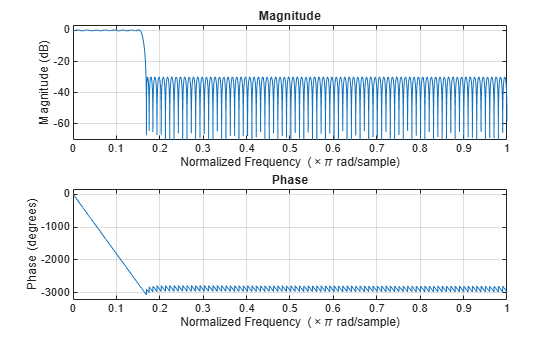```% Filter the signal to extract the component of interest rxWaveform = firFilter(demodulatedCC); % Plot the demodulated and filtered spectra filteredSpecPlot = ... hNRCarrierAggregationPlotSpectrum([demodulatedCC, rxWaveform],SR,... 'Demodulated and Filtered Waveform Power Spectrum',... {'Carrier aggregated signal' 'Filtered signal'});``````% Downsample the filtered carrier to its baseband rate rxWaveform = downsample(rxWaveform,OSRs(CCofInterest)/SRC);```

### EVM Measurements

The `hNRPDSCHEVM` helper function returns the PDSCH EVM by performing synchronization, OFDM demodulation, channel estimation, and equalization. The function displays the EVM for each slot and frame and the overall EVM averaged over the entire input waveform. The function also plots these graphs: EVM per OFDM symbol, slot, subcarrier, and overall EVM.

```% Parameterize the channel estimator configuration using the structure |cfg| cfg = struct(); cfg.Evm3GPP = true; % To measure EVM as defined in TS 38.104, Annex B(FR1) % / Annex C(FR2) set |Evm3GPP| to |true|. cfg.TargetRNTIs = []; cfg.PlotEVM = true; cfg.DisplayEVM = true; cfg.Label = wavegen{CCofInterest}.ConfiguredModel{1}; % Perform EVM measurements and plot results [evmInfo,eqSym,refSym] = hNRPDSCHEVM(wavegen{CCofInterest}.Config,... rxWaveform,cfg);```
```Low edge RMS EVM, Peak EVM, slot 1: 3.092 8.591% High edge RMS EVM, Peak EVM, slot 1: 2.795 8.020% Low edge RMS EVM, Peak EVM, slot 2: 2.996 8.556% High edge RMS EVM, Peak EVM, slot 2: 2.774 7.533% Low edge RMS EVM, Peak EVM, slot 3: 3.114 9.851% High edge RMS EVM, Peak EVM, slot 3: 2.796 7.604% Low edge RMS EVM, Peak EVM, slot 4: 2.972 8.648% High edge RMS EVM, Peak EVM, slot 4: 2.784 7.600% Low edge RMS EVM, Peak EVM, slot 5: 2.949 8.783% High edge RMS EVM, Peak EVM, slot 5: 2.774 7.659% Low edge RMS EVM, Peak EVM, slot 6: 3.079 9.422% High edge RMS EVM, Peak EVM, slot 6: 2.783 7.265% Low edge RMS EVM, Peak EVM, slot 10: 3.118 10.700% High edge RMS EVM, Peak EVM, slot 10: 2.779 8.086% Low edge RMS EVM, Peak EVM, slot 11: 3.070 9.473% High edge RMS EVM, Peak EVM, slot 11: 2.767 7.837% Low edge RMS EVM, Peak EVM, slot 12: 3.053 8.774% High edge RMS EVM, Peak EVM, slot 12: 2.780 7.284% Low edge RMS EVM, Peak EVM, slot 13: 2.993 8.781% High edge RMS EVM, Peak EVM, slot 13: 2.792 7.980% Low edge RMS EVM, Peak EVM, slot 14: 2.981 8.352% High edge RMS EVM, Peak EVM, slot 14: 2.789 7.437% Low edge RMS EVM, Peak EVM, slot 15: 2.930 8.303% High edge RMS EVM, Peak EVM, slot 15: 2.791 7.852% Low edge RMS EVM, Peak EVM, slot 16: 3.023 8.961% High edge RMS EVM, Peak EVM, slot 16: 2.788 7.686% Averaged low edge RMS EVM, frame 0: 3.029% Averaged high edge RMS EVM, frame 0: 2.784% Averaged RMS EVM frame 0: 3.029% ```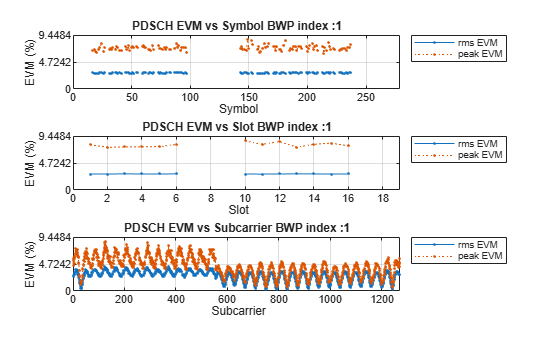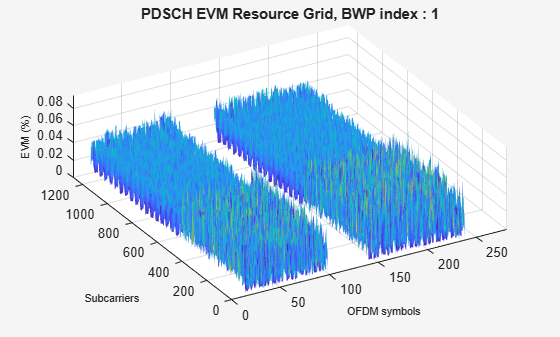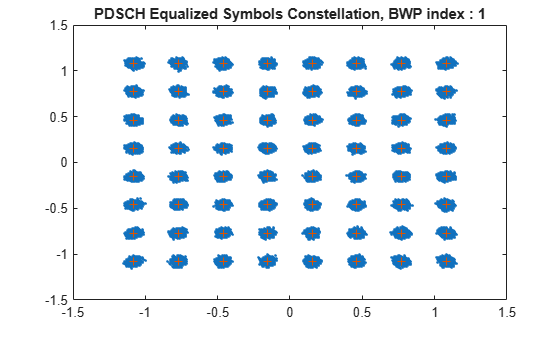```Averaged overall RMS EVM: 3.029% Overall Peak EVM = 10.7004% ```

### PDSCH Decoding

Decode the PDSCH of the recovered signal and check the resulting CRC for errors.

```% Perform time synchronization on the input waveform offset = nrTimingEstimate(carriers{CCofInterest},rxWaveform,... waveInfo{CCofInterest}.ResourceGridBWP); rxWaveform = rxWaveform(1+offset:end,:); % Perform OFDM demodulation rxGrid = nrOFDMDemodulate(carriers{CCofInterest},rxWaveform); % Get the allocated slots and OFDM symbols per slot allocatedSlots = zeros(1,size(resourcesInfo{CCofInterest}.WaveformResources.PDSCH.Resources,2)); for i=1:length(allocatedSlots) allocatedSlots(i) = resourcesInfo{CCofInterest}.WaveformResources.PDSCH.Resources(i).NSlot; end L = carriers{CCofInterest}.SymbolsPerSlot; % OFDM symbols per slot % Create a DLSCH decoder System object decodeDLSCH = nrDLSCHDecoder; decodeDLSCH.MultipleHARQProcesses = false; decodeDLSCH.TargetCodeRate = wavegen{CCofInterest}.Config.PDSCH{1}.TargetCodeRate; decodeDLSCH.LDPCDecodingAlgorithm = 'Layered belief propagation'; decodeDLSCH.MaximumLDPCIterationCount = 6; for NSlot=1:length(allocatedSlots) % Extract slot SlotID = allocatedSlots(NSlot); rxSlot = rxGrid(:,(1:L)+(SlotID*L),:); refSlot = waveInfo{CCofInterest}.ResourceGridBWP(:,(1:L)+(SlotID*L),:); % Perform channel estimation [estChannelGrid,noiseEst] = nrChannelEstimate(carriers{CCofInterest},... rxSlot,refSlot); % Get PDSCH resource elements from the received grid and channel estimate pdschIndices = resourcesInfo{CCofInterest}.WaveformResources.PDSCH.Resources(NSlot).ChannelIndices; [pdschRx,pdschHest] = nrExtractResources(pdschIndices,rxSlot,estChannelGrid); % Perform equalization [pdschEq,csi] = nrEqualizeMMSE(pdschRx,pdschHest,noiseEst); % Perform layer demapping, symbol demodulation, and descrambling modulation = wavegen{CCofInterest}.Config.PDSCH{1}.Modulation; RNTI = wavegen{CCofInterest}.Config.PDSCH{1}.RNTI; [dlschLLRs,rxSymbols] = nrPDSCHDecode(pdschEq,modulation,... carriers{CCofInterest}.NCellID,RNTI,noiseEst); % Scale LLRs by CSI csi = nrLayerDemap(csi); % CSI layer demapping NumCW = size(resourcesInfo{CCofInterest}.WaveformResources.PDSCH.Resources(NSlot).Codeword,2); for cwIdx = 1:NumCW Qm = length(dlschLLRs{cwIdx})/length(rxSymbols{cwIdx}); % Bits per symbol csi{cwIdx} = repmat(csi{cwIdx}.',Qm,1); % Expand by each bit per symbol dlschLLRs{cwIdx} = dlschLLRs{cwIdx} .* csi{cwIdx}(:); % Scaled symbols end % Calculate the transport block sizes for the codewords in the slot trBlkSize = resourcesInfo{CCofInterest}.WaveformResources.PDSCH.Resources(NSlot).TransportBlockSize; % Decode the DL-SCH transport channel decodeDLSCH.TransportBlockLength = trBlkSize; NLayers = wavegen{CCofInterest}.Config.PDSCH{1}.NumLayers; RVSequence = wavegen{CCofInterest}.Config.PDSCH{1}.RVSequence; [decbits,crc] = decodeDLSCH(dlschLLRs,modulation,NLayers,RVSequence); % Display the CRC status if crc disp(['Slot ' num2str(SlotID) ': CRC failed']); else disp(['Slot ' num2str(SlotID) ': CRC passed']); end end```
```Slot 1: CRC passed Slot 2: CRC passed Slot 3: CRC passed Slot 4: CRC passed Slot 5: CRC passed Slot 6: CRC passed Slot 10: CRC passed Slot 11: CRC passed Slot 12: CRC passed Slot 13: CRC passed Slot 14: CRC passed Slot 15: CRC passed Slot 16: CRC passed ```

### Bibliography

1. 3GPP TS 38.104 "NR; Base Station (BS) radio transmission and reception" 3rd Generation Partnership Project; Technical Specification Group Radio Access Network.

2. 3GPP TS 38.101-1 "NR; User Equipment (UE) radio transmission and reception; Part 1: Range 1 Standalone." 3rd Generation Partnership Project; Technical Specification Group Radio Access Network.

3. 3GPP TS 38.101-2 "NR; User Equipment (UE) radio transmission and reception: Part 2: Range 2 Standalone." 3rd Generation Partnership Project; Technical Specification Group Radio Access Network.

Download ebook